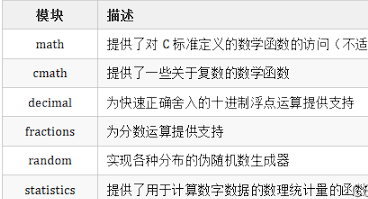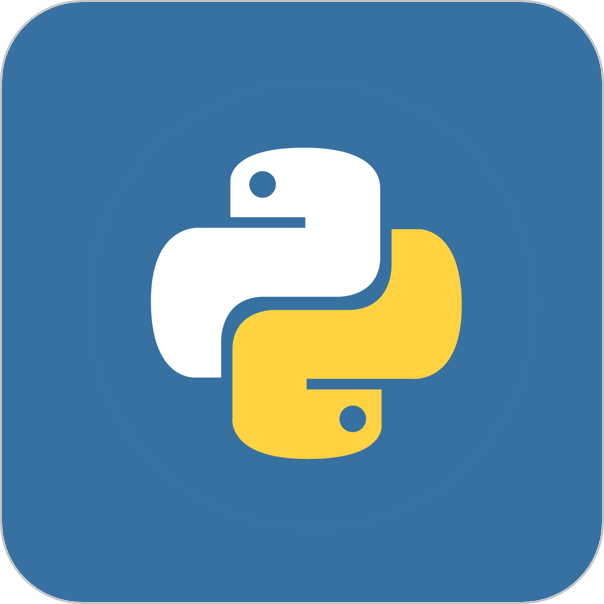## Python学习 -- Math模块和Random模块

math 模块提供了许多数学函数，用于执行各种数学运算。以下是一些常用的 math 函数以及相应的示例代码：math.sqrt(x): 计算平方根。import math x = 25 square_root = math.sqrt(x) print(f"√{x} = {square_root}")...

## Python基础 之 Python math 模块

Python基础 之 Python math 模块 Python math 模块 Python math 模块提供了许多对浮点数的数学运算函数。 math 模块下的函数，返回值均为浮点数，除非另有明确说明。 如果你需要计算复数，请使用 cmath 模块中的同名函数。 要使用 math 函数必须先导入...

101 课时 |
661 人已学 |

24 课时 |
1454 人已学 |

## 【科技少年】Python绘画编程第一课

20 课时 |
3313 人已学 |## 7.从入门到精通：Python pass 语句,Number 类型转换,math 模块、cmath 模块

Python pass 语句在 Python 中，pass 语句是一个空语句，它不做任何事情。在开发过程中，有时候需要定义一个占位符，但是又不想编写实际的代码，这时候可以使用 pass 语句来占位。以下是一个示例代码，展示了 pass 语句的用法：for i in range(1, 6): ...

## python——数学模块（math模块）（2）

pow(x, y)返回 x 的 y 次幂。看下示例：import math x = 3 y = 2 print(math.pow(x, y))fsum(iterable)返回迭代器中所有元素的和。看下示例：import math print(math.fsum((1, 2, 3, 4, 5)))gc...## Python中超好用的Math模块，高中知识一网打尽！

Math模块中，有很多基础的数学知识，我们必须要掌握的，例如：指数、对数、三角或幂函数等。因此，特意借着这篇文章，为大家讲价一些该库。由于该math模块与 Python 版本一起打包，因此您不必单独安装它，，直接导入：import mathmath模块常数P...

## python math模块

math模块函数： 函数（方法） 示例 说明 acos(x) 求x的反余弦（结果是弧度） acos(2.0)等于0.0 asin(x) 求x的反正弦（结果是弧度） asin(0.0)等于0.0 atan(x) 求x的反正切（结果是弧度） atan(0.0)等于0.0 ceil(x) 为x...

## python中math模块常用的方法整理

ceil:取大于等于x的最小的整数值，如果x是一个整数，则返回x copysign:把y的正负号加到x前面，可以使用0 cos:求x的余弦，x必须是弧度 degrees:把x从弧度转换成角度 e:表示一个常量 exp:返回math.e,也就是2.71828的x次方 expm1:返回math.e的x(...Python学习站
Python学习资料大全，包含Python编程学习、实战案例分享、开发者必知词条等内容。
672+人已加入

Python 脚本速查手册